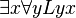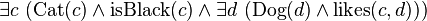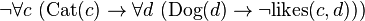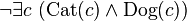# Predicate logic facts for kids

Kids Encyclopedia Facts

In logic and philosophy, predicate logic is a system of mathematical logic. It uses predicates to express the state of certain things, which are "incomplete propositions" with a placeholder for objects or subjects that must be inserted in order to obtain a valid proposition.

Predicate logic is different from propositional logic, in part because it has the concept of quantifiers. A quantifier is used in conjunction with a variable (say x) in order to talk about a general instance of x, and in doing so, this allows predicate logic to make statements about quantity.

The best-known quantifiers are the existential quantifier, represented by ∃, and the universal quantifier, represented by ∀. The existential quantifier is used to express statements of the form "there exists", and is true precisely when there is at least one mathematical object from the universe of discourse that matches the predicate or formula. On the other hand, the universal quantifier is used to express statements of the form "for all", and is true precisely when all possible mathematical objects of the universe of discourse match the specified predicate or formula.

In the notation of predicate logic, quantifiers directly precede (and thus introduce) variable names, which are then followed by other quantifiers or mathematical expressions, where the said variables are found. For example. one can use the expression$\exists x \forall y Lyx$ to mean "there is a person x such that for all persons y, y likes x" ("someone is liked by everyone.").

## Examples$\exist c\ (\text{Cat}(c) \and \text{isBlack}(c) \and \exist d\ (\text{Dog}(d) \and \text{likes}(c,d)))$

can be read as: "There is at least one cat which is black, and which likes (one or more) dogs."$\neg\forall c\ (\text{Cat}(c) \to \forall d\ (\text{Dog}(d) \to \neg\text{likes}(c,d)))$

can be read as: "It is not true that every cat doesn't like any dog."$\neg\exist c\ (\text{Cat}(c) \and \text{Dog}(c))$

can be read as: "There does not exist a cat which is also a dog."

## Related pagesPredicate logic Facts for Kids. Kiddle Encyclopedia.